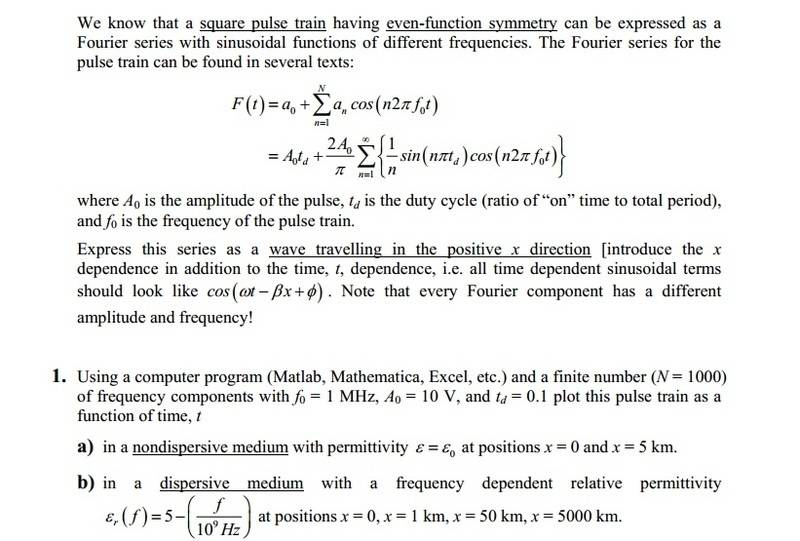# Square Pulse Train Fourier Series help?

• asdf12312
In summary, the speaker is asking for help with a problem involving a Square Pulse Train Fourier Series. They have attempted to change the equation to deal with the x-direction and have factored out the n in the cosine function. They also mention using Fourier series with MATLAB and finding the value of wavelength for β using the equation for phase velocity. The speaker later confirms that their Fourier series equation is 1+\frac{20}{\pi} \sum{\frac{1}{n}sin(\frac{n\pi}{10})cos[n(ωt-βx)]} where ω=2\pi*106, β=\frac{2\pi}{300} = \frac{\pi}{150}, and the series goes from

#### asdf12312

Square Pulse Train Fourier Series help??

## Homework Statement

problem+directions below:## Homework Equations

ω=2$\pi$f
β=$\frac{2\pi}{\lambda}$

## The Attempt at a Solution

Since the problem asks to make all time-dependent sinusoidal functions deal with x-direction, i don't think i need to worry about the sin function because it is dependent on td (duty cycle) and not time. I am aware of the cos format for x and t dependent but this is how i would plan to change it (for the equation with both sin and cos):
cos(n*2$\pi$f*t) -> cos[n(ωt-βx)]

basically I just factored out the n, and I don't think I need to include any phase shift. I just changed the cos part, the rest of the equation stays the same.

I can worry about using Fourier series with MATLAB later, I just wanted to know if the equation for the Fourier series I had was right. also, I don't know how to find the value of wavelength for β, since it is not given anywhere. would i need to use the equation for phase velocity, if it is related to the f given somehow?

Last edited:
OK, so thankfully our professor went over how to find the wavelength in a lecture. According to him in a non-dispersive medium, the phase velocity is simply c=speed of light=3x108. So if I use the equation up=f*λ and given f, then the wavelength λ=300m. So now my Fourier series equation looks like this:

1+$\frac{20}{\pi} \sum{\frac{1}{n}sin(\frac{n\pi}{10})cos[n(ωt-βx)]}$ where ω=2$\pi$*106, β=$\frac{2\pi}{300} = \frac{\pi}{150}$, and the Fourier series goes from n=1 to a finite number N=1000 (not infinity).

can someone confirm this is right so far?